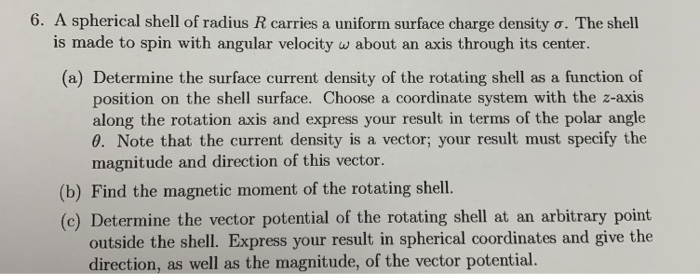# Question 6. A spherical shell of radius R carries a uniform surface charge density o. The shell is made to spin with angular velocity w about an axis through its center. (a) Determine the surface current density of the rotating shell as a function of position on the shell surface. Choose a coordinate system with the z-axis along the rotation axis and express your result in terms of the polar angle 0. Note that the current density is a vector; your result must specify the magnitude and direction of this vector. (b) Find the magnetic moment of the rotating shell. (c) Determine the vector potential of the rotating shell at an arbitrary point outside the shell. Express your result in spherical coordinates and give the direction, as well as the magnitude, of the vector potential.